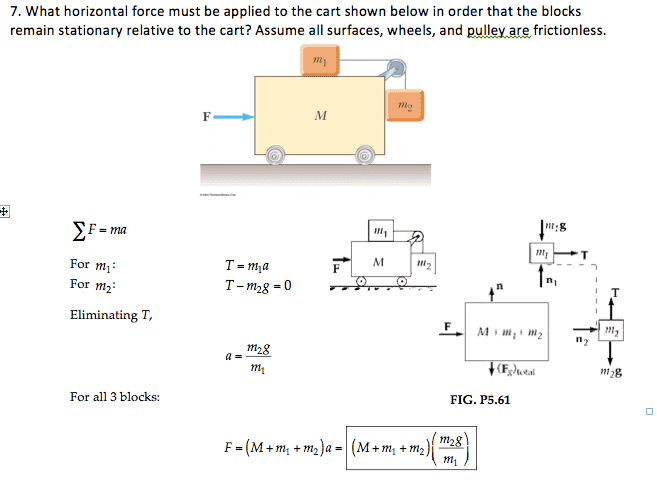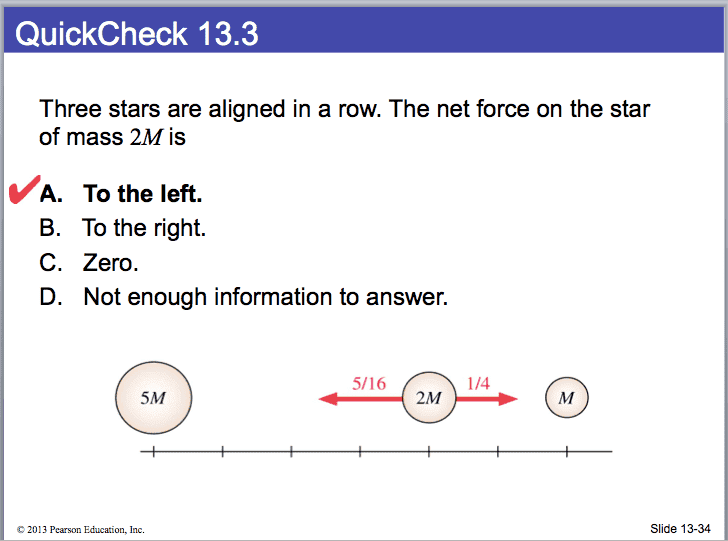# Dynamics Boxes 2

• yolo123

#### yolo123

Here is my method. Is it good?
T=m2g
m2g=m1a
a=m2g/m1
-n+Fpush=Ma. (n=normal force of m2 on M).
n=m2a=(m2)^2g/(m1a)

-n+Fpush=Ma.
Fpush=Ma+(m2)^2g/m

#### Attachments

•Screen Shot 2014-04-15 at 8.47.25 PM.png
16.9 KB · Views: 568

Here is my method. Is it good?
T=m2g
m2g=m1a
a=m2g/m1
-n+Fpush=Ma. (n=normal force of m2 on M).
n=m2a=(m2)^2g/(m1a)

-n+Fpush=Ma.
Fpush=Ma+(m2)^2g/m
No.

There is a horizontal force of T exerted to the left on M via the pulley.

There is also a downward force of T exerted on M, but that doesn't come into play here. It would if there was friction opposing the movement of M.Is the answer of the book wrong :O ?

Is the answer of the book wrong :O ?

Their result looks correct.

Perfect, I will come back to it!
I get -(5/8)M^2G/r^2 for the first attraction of the big mass and M^2G/(2r^2) for the attraction of the small mass.

Where did they get 5/16 and 1/4?
They merely used proportions.

Gravitational force is directly proportional to mass & inversely proportional to the square of distance.Ok perfect!

Coming back to the previous question:
Would the equation for M be:

-T+Fpush-n=Ma?

Why is there a T? :O Is the pulley not frictionless?

Ok perfect!

Coming back to the previous question:
Would the equation for M be:

-T+Fpush-n=Ma?

Why is there a T? :O Is the pulley not frictionless?

Yes, the equation is
-T+Fpush-n=Ma .​
Furthermore, T = m1a as well as m2 g .

Also, n = m2a .

Plugging those into -T+Fpush-n=Ma and solving for Fpush gives
Fpush = (M + m1 + m2)a​
in agreement with the book.

Yes, the pulley is frictionless, but there is thension T to the left on it an tension T downward on it. Both of these are transmitted to block M.